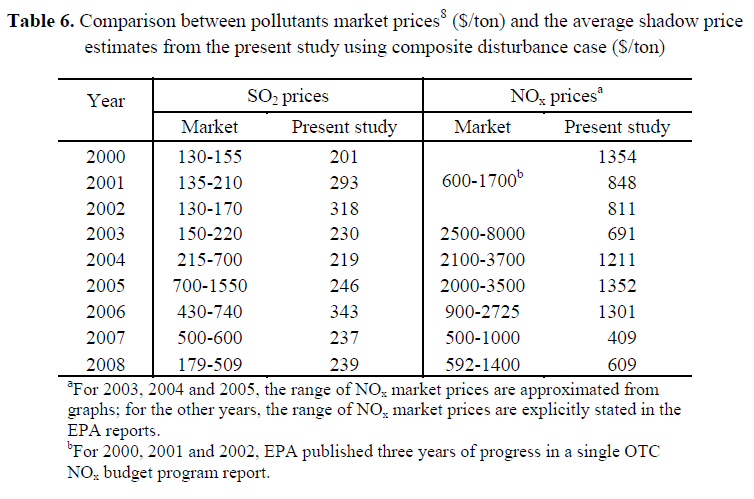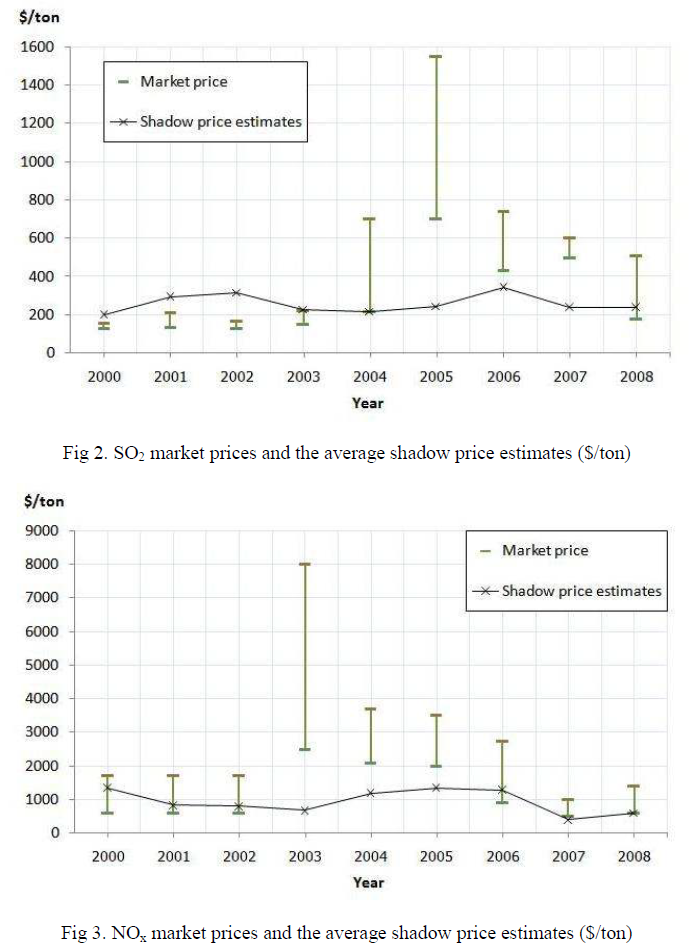.

Home

## Wednesday, June 6, 2012

### Estimating the shadow prices* of SO2 and NOx for U.S. coal power plants: A convex nonparametric least squares approach

http://dx.doi.org/10.1016/j.eneco.2012.01.002
Abstract: Weak disposability between outputs and pollutants, defined as a simultaneous proportional reduction of both outputs and pollutants, assumes that pollutants are byproducts of the output generation process and that a firm can “freely dispose” of both by scaling down production levels, leaving some inputs idle. Based on the production axioms of monotonicity, convexity and weak disposability, we formulate a convex nonparametric least squares (CNLS) quadratic optimization problem to estimate a frontier production function assuming either a deterministic disturbance term consisting only of inefficiency, or a composite disturbance term composed of both inefficiency and noise. The suggested methodology extends the stochastic semi-nonparametric envelopment of data (StoNED) described in Kuosmanen and Kortelainen (2011). Applying the method to estimate the shadow prices of SO2 and NOx generated by U.S. coal power plants, we conclude that the weak disposability StoNED method provides more consistent estimates of market prices.

#### Highlights

► Develops methodology to estimate shadow prices for SO2 and NOx in the U.S. coal power plants.
► Extends CNLS and StoNED methods to include the weak disposability assumption.
► Estimates the range of SO2 and NOx shadow prices as 201–343 \$/ton and 409–1352 \$/ton.
► StoNED method provides more accurate estimates of shadow prices than deterministic frontier.by Maethee Mekaroonreung and Andrew L. Johnson both of Department of Industrial and Systems Engineering, Texas A&M University, College Station, TX 77843-3131, USA
Energy EconomicsVolume 34, Issue 3; May, 2012; Pages 723–732
A full free version of the paper is currently available at http://ise.tamu.edu/ajohnson/CNLS_ShadowPrice.pdf
Keywords: Frontier estimation; Nonparametric regression; Parametric programming; Shadow pricing
* A shadow price can be defined as:
• The calculated price of a good or service for which no market price exists
• Opportunity cost of an activity or project to a society, computed where the actual price is not known or, if known, does not reflect the real sacrifice made.
• The assignment of dollar values to non-marketed goods such as production costs and intangible assets.
• The instantaneous change per unit of the constraint in the objective value of the optimal solution of an optimization problem obtained by relaxing the constraint. In other words, it is the marginal utility of relaxing the constraint, or, equivalently, the marginal cost of strengthening the constraint.
• The maximum price that management is willing to pay for an extra unit of a given limited resource. For example, if a production line is already operating at its maximum 40-hour limit, the shadow price would be the maximum price the manager would be willing to pay for operating it for an additional hour, based on the benefits he would get from this change.
• More formally, the shadow price is the value of the Lagrange multiplier at the optimal solution, which means that it is the infinitesimal change in the objective function arising from an infinitesimal change in the constraint. This follows from the fact that at the optimal solution the gradient of the objective function is a linear combination of the constraint function gradients with the weights equal to the Lagrange multipliers. Each constraint in an optimization problem has a shadow price or dual variable.
When performing different types of cost-benefit analyses, certain costs or benefits are intangible and, in order to fully analyze a scenario, all of these variables must be assigned values. For example, when performing a cost-benefit analysis on a mining operation, the lost intangible value associated with the scenic views must be priced and factored in as a cost.